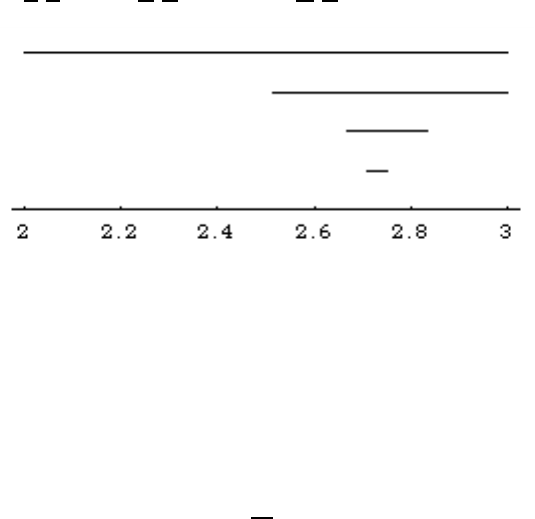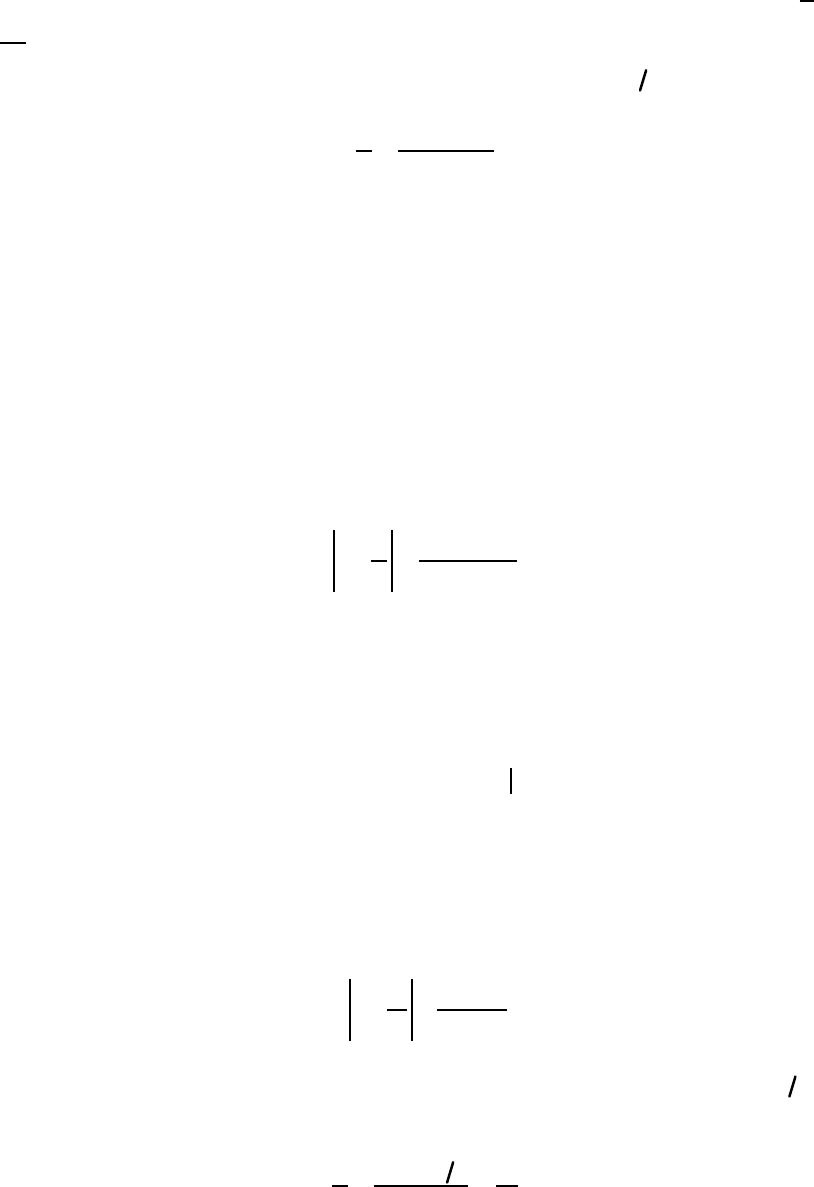The Euler's number was first introduced by Jacob Bernoulli in 1683....
Sondow starts by defining an interval $I_1$ of length 1. The interv...
Now Sondow starts with $I_1 = [2,3]$ and inductively constructs $I_... Euler's number is just the intersection of all the intervals$I_n$.... Since$e$is the intersection point of all the intervals$I_n$it h... Shouldn't the proof of (6) read "Since e lies in the interior of th...A Geometric Proof that e is Irrational and a New Measure of its Irrationality Jonathan Sondow 1. INTRODUCTION. While there exist geometric proofs of irrationality for 2 , , no such proof for e, π , or ln 2 seems to be known. In section 2 we use a geometric construction to prove that e is irrational. (For other proofs, see [1, pp. 27-28], [3, p. 352], , [10, pp. 78-79], [15, p. 301], , [17, p. 11], , , and [21, p. 302].) The proof leads in section 3 to a new measure of irrationality for e, that is, a lower bound on the distance from e to a given rational number, as a function of its denominator. A connection with the greatest prime factor of a number is discussed in section 4. In section 5 we compare the new irrationality measure for e with a known one, and state a number- theoretic conjecture that implies the known measure is almost always stronger. The new measure is applied in section 6 to prove a special case of a result from , leading to another conjecture. Finally, in section 7 we recall a theorem of G. Cantor that can be proved by a similar construction. 2. PROOF. The irrationality of e is a consequence of the following construction of a nested sequence of closed intervals I n . Let I 1 = [2, 3] . Proceeding inductively, divide the interval I n 1 into n ( 2) equal subintervals, and let the second one be I n (see Figure 1). For example, I 2 = 5 2! , 6 2! [ ] , , and I 4 = 65 4! , 66 4! [ ] . Figure 1. The intervals I 1 , I 2 , I 3 , I 4 . The intersection n =1 I n = {e} (1) is then the geometric equivalent of the summation (see the Addendum) 1 n! n =0 = e . (2)2 When n > 1 the interval I n +1 lies strictly between the endpoints of I n , which are a n! and a +1 n! for some integer a = a(n) . It follows that the point of intersection (1) is not a fraction with denominator n! for any n 1 . Since a rational number p q with q > 0 can be written p q = p (q 1)! q! , (3) we conclude that e is irrational. Question. The nested intervals I n intersect in a number—let's call it b. It is seen by the Taylor series (2) for e that b = e . Using only standard facts about the natural logarithm (including its definition as an integral), but not using any series representation for log, can one see directly from the given construction that log b = 1 ? 3. A NEW IRRATIONALITY MEASURE FOR e. As a bonus, the proof leads to the following measure of irrationality for e. Theorem 1. For all integers p and q with q > 1 e p q > 1 (S(q) + 1)! , (4) where S(q) is the smallest positive integer such that S(q)! is a multiple of q. For instance, S(q) = q if 1 q 5 , while S(6) = 3 . In 19l8 A. J. Kempner  used the prime factorization of q to give the first algorithm for computing S(q) = min {k > 0 : q k!} (5) (the so-called Smarandache function ). We do not use the algorithm in this note. Proof of Theorem 1. For n > 1 the left endpoint of I n is the closest fraction to e with denominator not exceeding n!. Since e lies in the interior of the second subinterval of I n , e m n! > 1 (n + 1)! (6) for any integer m. Now given integers p and q with q > 1 , let m = pS(q)! q and n = S(q) . In view of (5), m and n are integers. Moreover, p q = pS(q)! q S(q)! = m n! . (7) Therefore, (6) implies (4). Shouldn't the proof of (6) read "Since e lies in the interior of the second subinterval of I_{n+1} " ? The interval$I_n$has length$\frac1{n!}$, but each of its subintervals have length$\frac1{(n+1)!}$. Since$e$is the intersection point of all the intervals$I_n$it has to be inside all the intervals and cannot be one of the extremes$\frac{a_n}{n!}$or$\frac{a_n+1}{n!}$. Now if we assume that$e$is rational it can be written in the form$e=\frac{p}{q}$where$p$and$q$are integers and so$e=\frac{p}{q}=\frac{p(q-1)!}{q(q-1)!}=\frac{p(q-1)!}{q!}$Since$p(q-1)!$is also an integer it means that$e$can be written in the form of an extreme$\frac{p(q-1)!}{q!} \equiv \frac{a_n}{n!}$of a certain interval$I_n$. This contradiction means that$e$must be an irrational. Sondow starts by defining an interval$I_1$of length 1. The interval$I_n$is obtained by dividing the previous interval$I_{n-1}$into$n$intervals of the same length, so that the length of$I_n$will be$\frac{1}{n!}$. Looking at Fig. 1 we can see that the size of$I_1$is 1 and the size of the interval below$I_2$is$\frac{1}{2!}=0.5$. Euler's number is just the intersection of all the intervals$I_n$. As can be seen in Fig. 1 the larger the$n$the closer will be the extremes of the interval to$e$. Now Sondow starts with$I_1 = [2,3]$and inductively constructs$I_n$by splitting$I_{n-1}$into$n$intervals of the same length. \begin{eqnarray*} & I_1 &=[1+\frac{1}{1!},1+\frac{2}{1!}]=[2,3] \\ & I_2 &=[1+\frac{1}{1!}+\frac{1}{2!},1+\frac{1}{1!} +\frac{2}{2!}]=[\frac{5}{2!},\frac{6}{2!}] \\ & I_3 &=[1+\frac{1}{1!}+\frac{1}{2!} +\frac{1}{3!},1+\frac{1}{1!} + \frac{1}{2!}+\frac{2}{3!}] \\ &=&[\frac{16}{3!},\frac{17}{3!}] \end{eqnarray*} Which means that$I_n$starts in$1+\frac{1}{1!}+\frac{1}{2!}+...+\frac{1}{n!}=\frac{a_n}{n!}$and, since the length is$\frac{1}{n!}$, ends in$\frac{a_n+1}{n!}$.$I_n=[\frac{a_n}{n!},\frac{a_n+1}{n!}]$where$a_n$is an integer. The Euler's number was first introduced by Jacob Bernoulli in 1683. Euler was the first person to prove that$e$was irrational in 1737 using a simple continued fraction (here is a link to Euler's original [proof](https://math.dartmouth.edu/~euler/docs/originals/E071.pdf)). Fourier later established one of the most well-known proofs that$e$is irrational based on the inequality$e = \sum_{n=0}^{\infty}\frac{1}{n!} $. However, in this paper J. Sondow is the first to use geometrical arguments to prove that$e\$ is irrational.